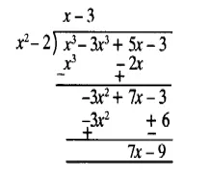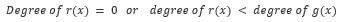# Chapter 2 Polynomials

At Saralstudy, we are providing you with the solution of Class 10 Mathematics Polynomials according to the latest NCERT (CBSE) Book guidelines prepared by expert teachers. Here we are trying to give you a detailed answer to the questions of the entire topic of this chapter so that you can get more marks in your examinations by preparing the answers based on this lesson. We are trying our best to give you detailed answers to all the questions of all the topics of Class 10th mathematics Polynomials so that you can prepare for the exam according to your own pace and your speed.

Download pdf of NCERT Examplar with Solutions for Class Mathematics Chapter 2 Polynomials

### Exercise 1 ( Page No. : 28 )

•  Q1 The graphs of y = p(x) are given in Fig. 2.10 below, for some polynomials p(x). Find the number of zeroes of p(x), in each case. Ans: Total no of zeroes of a polynomial equation = the number of times the curve intersect x-axis In this graph the number of zeroes of p(x) is 0, because the graph is parallel to x- axis and does not intersect at any point on x-axis. In this graph the number of zeroes of p(x) is 1, because the curve intersects x-axis only at one point. In this graph the number of zeroes of p(x) is 3, because the curve intersects x-axis at three points.  In this graph the number of zeroes of p(x) is 2, because the curve intersects x-axis at two points. In this graph the number of zeroes of p(x) is 4, because the curve intersects x-axis at four points. In this graph the number of zeroes of p(x) is 3, because the curve intersects x-axis at three points.

### Exercise 2 ( Page No. : 33 )

•  Q1 Find the zeroes of the following quadratic polynomials and verify the relationship between the zeroes and the coefficients. (i) x2 – 2x – 8 (ii) 4s2 – 4s + 1 (iii) 6x2 – 3 – 7x (iv) 4u2 + 8u (v) t2 – 15 (vi) 3x2 – x – 4 Ans: (i)   x2 – 2x – 8                  = x – 4x + 2x – 8                                   = x(x – 4) + 2(x – 4)                  = (x + 2) (x – 4)                 The value of x2 – 2x – 8 is zero if (x + 2) = 0 and (x – 4) = 0                       x = -2 or   x = 4                        Sum of zeroes = (-2 + 4) = 2 = - coefficient of x                                                                         coefficient of x2                              Product of zeroes = (-2) × 4 = -8 = Constant term                                                                              coefficient of x2 (ii)          4s2 – 4s + 1                           = 4s2 – 2s – 2s + 1                         = 2s (2s – 1) – 1 (2s – 1)                          = ( 2s – 1 )  ( 2s – 1 )                            The value of 4s2 – 4s + 1 is zero , if (2s-1) = 0 and (2s-1 ) = 0                        s = 1/2 , 1/2                        Sum of zeroes = (1/2 + 1/2) = 1   - coefficient of x                                                                             coefficient of x2                       Product of zeroes =1/2 × 1/2 = 1/4 =  constant term                                                                                 coefficient of x2              (iii)    6x2 –7x – 3                           = 6x – 9x + 2x – 3                                           = 3x (2x – 3) + 1(2x – 3)                                              = (3x + 1) (2x – 3)                                      The value of  6x2 –7x – 3 is zero, if (3x + 1) = 0 and (2x – 3) = 0                             X = -1 /3 , 3/2                             Sum of zeroes = ( -1/3 + 3/2) = 7/6 =  - coefficient of x                                                                                         coefficient of x2                            Product of zeroes = -1/3 × 3/2 = -3/2 =  constant term                                                                                         coefficient of x2             (iv)        4u2+8u                        4u(u+2)                        The value of 4u2+8u is zero, if 4u = 0 and (u+2) =0                         u   = 0,  - 2                        Sum of zeroes = ( 0+ (-2)) = -2 =  - coefficient of x                                                                                coefficient of x2                          Product of zeroes = (-2) × 0 = 0 =   constant term                                                                                  coefficient of x2             (v)(vi)  3x2–x–4                             3x – 4x + 3x – 4                        =  x (3x – 4) + 1 (3x – 4)                        The value of 3x – x + 4 is zero, if (3x – 4) = 0 and (x + 1) = 0                              Sum of zeroes = [4/3 + ( -1)] = 1/3 = - coefficient of x                                                            coefficient of x2                           Product of zeroes = (-1) × 4/3 = -4/3 = constant term                                                                                           coefficient of x2 Q2 Find a quadratic polynomial each with the given numbers as the sum and product of its zeroes respectively. Ans: (i) Let α, β are the zeroes of the polynomial ax2+ bx +c, therefore                                Sum of zeroes (α + β) = 1/4 =  -b/a                             Product of zeroes (αβ) = -1 = c/a                             On comparing,                              a = 4, b = -1 and c = - 4                          Hence, the required polynomial is 4x2 –x – 4 (ii)(iii)  Let α, β are the zeroes of the polynomial ax2+ bx +c, therefore                                 Sum of zeroes (α + β) = 0 = -b/a                          Product of zeroes (αβ) = √5 = √5/1 = c/a                       On comparing,                         a = 1, b = 0 and c = √5                        Hence, the required polynomial is x2 + √5### Exercise 3 ( Page No. : 36 )

•  Q1 Divide the polynomial p(x) by the polynomial g(x) and find the quotient and remainder in each of the following : (i) p(x) = x3 – 3x2 + 5x – 3, g(x) = x2 – 2    (ii) p(x) = x4 – 3x2 + 4x + 5, g(x) = x2 + 1 – x      (iii) p(x) = x4 – 5x + 6, g(x) = 2 – x2 Ans: (i) Given ,                      Dividend = p(x) = x2 – 3x2 + 5x – 3                          Divisor = g(x) = x2 – 2Quotient =  x – 3                              Reminder = 7x – 9 (ii) Given,                            Dividend  = p(x) = x4 – 3x2  + 4x + 5                           Divisor = g(x) = x2 + 1 – xAs we can see the remainder is 0. Thereofre we can say that  first  polynomial is a factor of second polynomial. (ii) Given,    First polynomial = x2+3x+1    Second polynomial = 3x4 + 5x3 – 7x2 + 2x + 2Therefore ,  g(x) = ( x2 – x + 1 ) Q5 Give examples of polynomials p(x), g(x), q(x) and r(x), which satisfy the division algorithm and (i) deg p(x) = deg q(x) (ii) deg q(x) = deg r(x) (iii) deg r(x) = 0 Ans: we know that(i) Deg P(x) = deg g (x)     The degree of dividend or quotient can be equal, only if the divisor is a constant (degree 0)     Then, let p(x) = 3x2 –  6x + 5      Let g(x) = 3      Therefore,  q(x) = x2 – 2x +1  and r(x) = 2 (ii) Deg  q(x)  =  deg r(x)          Let p(x) = x2 + 1      Let g(x) = x      Therefore, q(x) = x + 1 and r(x) = 0      Here, we can see the degree of quotient is equal to the degree of remainder.      Hence, division algorithm is satisfied here.    (iii)    deg r(x) = 0 The degree of remainder is zero, only if the remainder left after division algorithm is Constant. Let p(x) = x2 + 1 Let g(x) = x                 Therefore, q(x)= x  and r(x) = 1  Here we can see the degree of remainder is zero. Hence division algorithm is satisfied here.

### Exercise 4 ( Page No. : 37 )

•  Q1 Verify that the numbers given alongside of the cubic polynomials below are their zeroes. Also verify the relationship between the zeroes and the coefficients in each case: Ans: (i)                         Here f(x) = 2x³ + x² - 5x + 2                             Given roots of f(x) are ½, 1, -2 F(1/2) = 2×(1/2)³ + (1/2)² - 5(1/2 ) + 2 = 0 F(1) = 2(1)³ + 1² - 5(1) + 2 = 0 F(-2) = 2(-2)³ + (-2)² - 5(-2) + 2 = 0 Hence, ½, 1 and -2 are the zeroes of f(x). Therefore, sum of zeroes = -b/a -1/2 Sum of product of zeroes taken two at a time = c/a = -5/2 Product of zeroes = -d/a = 2 (ii)                    Let the f(x) = ax³ + bx² + c + d Let α, β and γ be the zeroes of the polynomial f(x). Then, sum of zeroes = -b/a = 2/1 ………………(i) Sum of product of zeroes taken two at a time = c/a = -7. ………………..(ii) Product of zeroes = -d/a = -14 ……………….(iii) From equation (i), (ii) and (iii) we have a = 1 , b = -2 , c = -7 and d = 14 Therefore the required polynomial on putting the values of a, b, c and d F(x) = x³ - 2x² - 7x + 14 Q2 Find a cubic polynomial with the sum, sum of the product of its zeroes taken two at a time, and the product of its zeroes as 2, –7, –14 respectively. Ans: Let the p(x) = ax3+bx2+ cx + d                             Sum of zeroes and α, β and γ be the zeroes.                             Then, α, β and γ = -b/ a = 2/1    …………………..(i)                             αβ + βγ + γα= c/a = -7      …………………….(ii) ‘                           αβγ = -d /a = -14               ……………………………..(iii)                             From equation (i), (ii) and (iii), we get                             a = 1, b = -2, c = -7 and d = 14                             Therefore, the required polynomial on putting the value of a, b, c and d is P(x) = x3 - 2x2 – 7x + 14 Q3 If the zeroes of the polynomial x3 – 3x2 + x + 1 are a – b, a, a + b, find a and b. Ans: Given, p(x) = x3 - 3x2 + x + 1 And zeroes are given as a – b, a, a + b Now, comparing the given polynomial with general expression, we get; ∴ ax3+bx2+ cx + d = x3 – 3x2+ x + 1 a = 1, b = -3, c = 1 and d = 1 Sum of zeroes = a – b + a + a + b -b/a = 3a Putting the values b and a - (-3)/1 = 3a                 a = 1 Thus, the zeroes are 1 - b, 1, 1 + b. Now, product of zeroes = 1(1 – b) (1 + b) d/a = 1 - b2 -1/1 = 1- b2 b2 = 1 + 1 = 2 b = √2 Hence, 1, -√2, 1, 1 + √2 are the zeroes of x3 – 3x2 + x + 1 Q4 Ans:Therefore, By equating the remainder with zero, we have (-10 + 2k) = 0 => 2k = 10  => k = 5 Or, 10 – a – 8k + k2 = 0 Putting the value of k, we get 10 – a – 8(5) + (5)2 = 0 10 – a – 40 + 25 = 0 - a – 5 = 0 => a = -5 Hence, k = 5 and a = -5